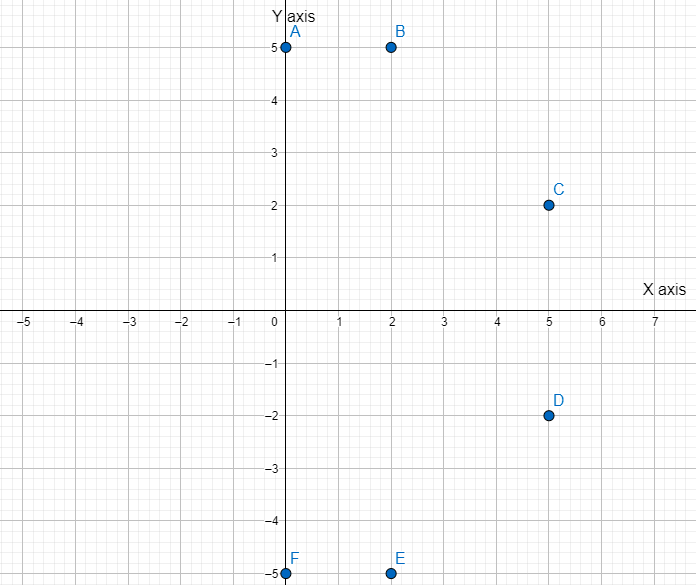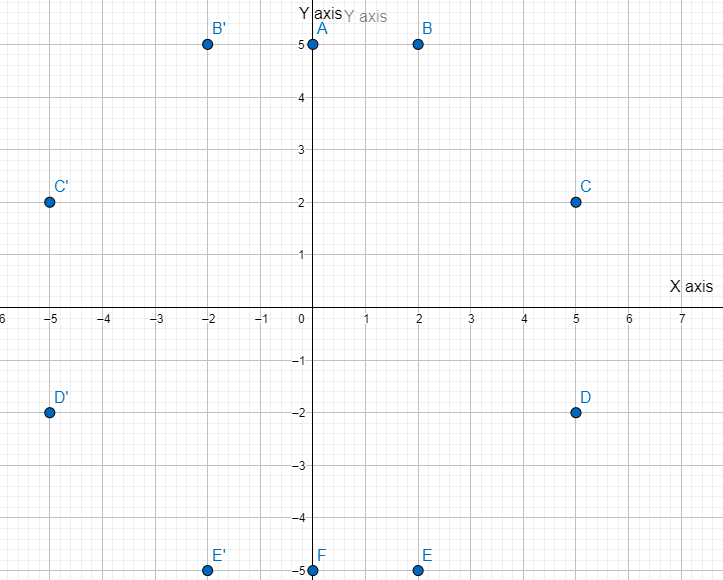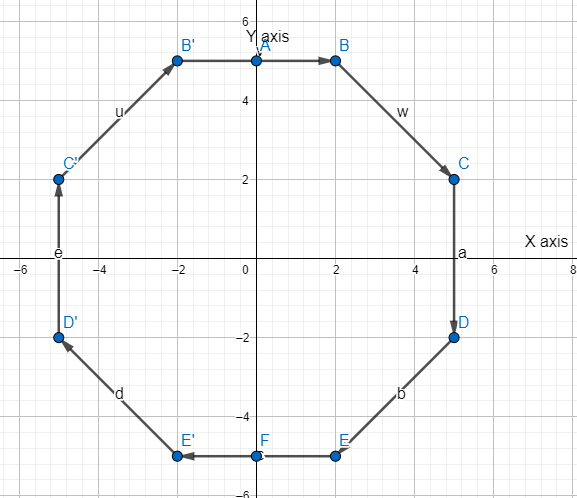Courses
Courses for Kids
Free study material
Free LIVE classes
MoreLIVE
Join Vedantu’s FREE Mastercalss

# Use a graph paper for this question taking 1cm=1 unit along both the x and y axis:(i) Plot the points A(0,5), B(2,5), C(5,2), D(5,−2), E(2,−5) and F(0,−5).(ii) Reflect the points B,C,D and E on the y-axis and name them respectively as B′,C′,D′ and E′.(iii) Write the coordinates of B′,C′,D′ and E′.(iv) Name the figure formed by BCDEE′D′C′B′.(v) Name a line of symmetry for the figure formed.Verified
360k+ views
Hint: For solving this question, we should be aware about the basics of graph plotting. X-direction means going towards right while Y-direction means going upwards. Further, reflecting a point on the axis means finding a point that is at the same distance from the axis as the original point but on the opposite side.

(i) Before beginning, we note that coordinates are represented as (x,y), where x is the x coordinate and y is the y coordinate. By default, we take 1 unit on the graph as 1 cm.
By noting that positive value of x coordinate means moving towards right by the respective unit, negative value of x coordinate means moving towards left by the respective unit, positive value of y coordinate means moving towards up by the respective unit, negative value of y coordinate means moving towards down by the respective unit. Thus, keeping this in mind, we can plot the given points. We have,(ii) Now, reflecting an object means finding a point that is at the same distance from the axis as the original point but on the opposite side. Thus, for example for B (2,5), we would have B’ (-2,5) as the reflected point (when reflected from y axis) since this would be equidistant from y-axis as B points. Thus, doing this for all points, we have,(iii) The coordinates of B’ is (-2,5), C’ is (-5,2), D’ is (-5,-2) and E’ is (-2,-5). This can be read from the graph for the points B’, C’, D’ and E’. For example, B’ is 2 units left (so x coordinate is -2) and 5 units upwards (so y coordinate is +5).
(iv) For convenience, this figure is drawn on the graph as shown below,(v) The line of symmetry basically means the line which can divide a figure into symmetric portions. To name one line of symmetry, we have x axis, to make it clear see the figure above in (iv). We can see the upper half figure and the lower half figure are symmetric.

Note: Plotting on the graph is generally helpful in various questions like those involving geometry where plotting on a graph paper can greatly simplify the understanding of the geometry problem. Further, while understanding reflection, this is similar to the reflection in a mirror (here the respective axis acts as the mirror). Thus, the reflected object should be at the same distance from the axis as is the original object from the axis but on the opposite side.

Last updated date: 21st Sep 2023
Total views: 360k
Views today: 5.60k